\$intHour = \$hour; if ((\$intHour >= 0) && (\$intHour < 12)) { \$strAMPM = "AM"; }else{ \$intHour = \$intHour - 12; \$strAMPM = "PM"; } \$strDateFormat = \$arr_zero[strlen(strval(\$day))].\$day."/".\$arr_zero[strlen(strval(\$month))].\$month."/".substr(\$int_year_date,-2)." - ".\$arr_zero[strlen(strval(\$intHour))].\$intHour& ":".\$arr_zero[strlen(strval(\$minute))].\$minute." ".\$strAMPM; break; case "dmmyy" : \$strDateFormat = \$day." ".\$arr_fullmonth[\$month - 1]." ".substr(\$int_year_date,-2); break; case "dmmyyyy" : \$strDateFormat = \$day." ".\$arr_fullmonth[\$month - 1]." ".\$int_year_date; break; case "d/mm/yyyy" : \$strDateFormat = \$day."/".\$arr_month[\$month-1]."/".\$int_year_date; break; default : \$strDateFormat = \$arr_zero[strlen(strval(\$day))].\$day."/".\$arr_month[\$month-1]."/".substr(\$int_year_date,-2); } }else{ \$strDateFormat = "-"; } \$RLDateFormat = \$strDateFormat; return \$RLDateFormat; } function StrToDateSerial(\$dte_date){ if (trim(\$dte_date) == "" ){ \$dte_temp = ""; }else{ \$dte_temp = \$dte_date; \$arr_date_part = split("-", \$dte_temp); \$dte_temp = date("Y-m-d", mktime(0, 0, 0, intval(trim(\$arr_date_part)) ,intval(trim(\$arr_date_part)), intval(trim(\$arr_date_part))) ); } \$StrToDateSerial = \$dte_temp; return \$StrToDateSerial; } function StrToDateSerial_3(\$dte_date){ if (trim(\$dte_date) == "" ){ \$dte_temp = ""; }else{ \$dte_temp = \$dte_date; \$arr_date_part = split("/", \$dte_temp); \$dte_temp = date("Y-m-d", mktime(0, 0, 0, intval(trim(\$arr_date_part)) ,intval(trim(\$arr_date_part)), intval(trim(\$arr_date_part))) ); } \$StrToDateSerial = \$dte_temp; return \$StrToDateSerial; } function DateFormat(\$str_date , \$int_lang){ //Display on Document if (\$str_date != "") { \$dte=strpos(\$str_date," "); if (\$dte != 0) { \$dte=substr(\$str_date, 0,\$dte); \$time=strrpos(\$str_date," "); \$time=substr(\$str_date, \$time); list(\$year, \$month, \$day) = explode("-", \$dte); list(\$hour, \$minutes, \$seconds) = explode(":", \$time); }else{ \$dte=\$str_date; list(\$year, \$month, \$day) = explode("-", \$dte); } if (\$int_lang == 1) { \$arr_month = array("Jan","Feb","Mar", "Apr" , "May" , "Jun" , "Jul" , "Aug" , "Sep", "Oct" , "Nov","Dec"); }else{ \$arr_month = array("┴.ż.","Ī.Š.","┴š.ż.", "Ó┴.┬." , "Š.ż." , "┴į.┬." , "Ī.ż." , "╩.ż." , "Ī.┬.", "Ą.ż." , "Š.┬.","Ė.ż.") ; } \$arr_zero = array("0",""); \$int_year_date = \$year; if (\$int_lang == 1) { \$int_year_date = \$int_year_date; \$DateFormat = \$arr_zero[strlen(strval(\$day))].\$day."/".\$arr_month[\$month-1]."/".substr(\$int_year_date,-2); }else{ \$int_year_date = \$int_year_date + 543; \$DateFormat = \$arr_zero[strlen(strval(\$day))].\$day." ".\$arr_month[\$month-1]." ".substr(\$int_year_date,-2); } }else{ \$DateFormat = "-"; } return \$DateFormat; } function DateValueFormat(\$str_date){ \$dte=strpos(\$str_date," "); if (\$dte != 0) { \$dte=substr(\$str_date, 0,\$dte); \$time=strrpos(\$str_date," "); \$time=substr(\$str_date, \$time); list(\$year, \$month, \$day) = explode("-", \$dte); list(\$hour, \$minutes, \$seconds) = explode(":", \$time); }else{ \$dte=\$str_date; list(\$year, \$month, \$day) = explode("-", \$dte); } \$arr_zero = array("0",""); \$DateValueFormat = \$day."-".\$month."-". \$year; return \$DateValueFormat; } function DateValueFormat_3(\$str_date){ \$dte=strpos(\$str_date," "); if (\$dte != 0) { \$dte=substr(\$str_date, 0,\$dte); \$time=strrpos(\$str_date," "); \$time=substr(\$str_date, \$time); list(\$year, \$month, \$day) = explode("-", \$dte); list(\$hour, \$minutes, \$seconds) = explode(":", \$time); }else{ \$dte=\$str_date; list(\$year, \$month, \$day) = explode("-", \$dte); } \$arr_zero = array("0",""); \$DateValueFormat3 = \$day."/".\$month."/". \$year; return \$DateValueFormat3; } function getDayOfMonth( \$int_month, \$int_year ){ \$DOMonth = array( 31, 28, 31, 30, 31, 30, 31, 31, 30, 31, 30, 31 ); \$LDOMonth = array( 31, 29, 31, 30, 31, 30, 31, 31, 30, 31, 30, 31); if ( (\$int_year % 4) == 0 ) { if ( (\$int_year % 100 == 0) && (\$int_year % 400 != 0) ){ \$getDayOfMonth = \$DOMonth[\$int_month-1]; } \$getDayOfMonth = \$LDOMonth[\$int_month-1]; }else{ \$getDayOfMonth = \$DOMonth[\$int_month-1]; } return \$getDayOfMonth; } function getDateTime(\$intDay, \$intMonth, \$intYear, \$intHour, \$intMinute, \$strPeriod){ \$strDateTime = \$intMonth."/".\$intDay."/".\$intYear." ".\$intHour.":".\$intMinute.":00 ".\$strPeriod; \$getDateTime = \$strDateTime; return \$getDateTime; } ?> '~]+>~si', 1 => '~]+>~si', 2 => '~]+>~si', 3 => '~]+>~si', 4 => '~]+>~si', 5 => '~]+>~si', 6 => '~]+>~si', 7 => '~]+>~si', ); \$html = preg_replace(\$tags,"\n",\$html); \$html = preg_replace('~\s*]+>~si',' - ',\$html); \$html = preg_replace('~<[^>]+>~s','',\$html); // reducing spaces \$html = preg_replace('~ +~s',' ',\$html); \$html = preg_replace('~ ~',' ',\$html); \$html = preg_replace('~^\s+~m','',\$html); \$html = preg_replace('~\s+\$~m','',\$html); // reducing newlines \$html = preg_replace('~\n+~s',"\n",\$html); return \$html; } function genPageNumber(\$strUrl, \$intPage, \$intTotalPage, \$intTotalRow, \$bitSelectAll , \$intPagePerOnce){ \$strPage = ""; \$intPage = intval(\$intPage); //------- Set << mark ------ if (\$bitSelectAll==true) { \$strPage = "Showing All [Total ".\$intTotalRow."]"; //echo \$strPage; }else{ //--- Page per Once --- \$intTotalRange = floor(intval( \$intTotalPage / \$intPagePerOnce )); if ((\$intTotalPage % \$intPagePerOnce) > 0) { \$intTotalRange = \$intTotalRange + 1;} \$intCurrentRange = floor(intval( \$intPage / \$intPagePerOnce )); if ((\$intPage % \$intPagePerOnce) > 0) { \$intCurrentRange = \$intCurrentRange + 1;} if (\$intCurrentRange <= 0) { \$intCurrentRange = 1;} \$intStartPage = (( \$intCurrentRange - 1 ) * \$intPagePerOnce ) + 1; \$intEndPage = \$intStartPage + \$intPagePerOnce - 1; //--- [Next Page Number] --- \$intNextFromPage = \$intEndPage + 1; \$intNextToPage = \$intNextFromPage + \$intPagePerOnce - 1; if (\$intNextToPage > \$intTotalPage) { \$intNextToPage = \$intTotalPage;} //--- [Previous Page Number] --- \$intPrevFromPage =\$intStartPage - \$intPagePerOnce; \$intPrevToPage = \$intPrevFromPage + \$intPagePerOnce - 1; \$intParamPosition = strpos(\$strUrl, "?"); if (\$intParamPosition > 0) { \$strParamSymbol = "&"; }else{ \$strParamSymbol = "?"; } if (\$intCurrentRange > 1 ) { \$strPageNumber = "<< ". \$intPrevFromPage."-".\$intPrevToPage." | "; } for (\$iPage = \$intStartPage; \$iPage<=\$intEndPage; \$iPage++){ if (\$intTotalPage >= \$iPage) { \$strPageLink = "".\$iPage.""; \$strPageNumber = \$strPageNumber.\$strPageLink." | "; } } if ( \$intTotalRange > 1 ) { if (\$intCurrentRange < \$intTotalRange) { //------- Set >> mark ------ if (\$intNextFromPage < \$intNextToPage) { \$strToPage = \$intNextFromPage."-".\$intNextToPage; }else{ \$strToPage = \$intNextFromPage; } \$strPageNumber = \$strPageNumber."".\$strToPage." >> "; } } if (\$intTotalPage > 1) { //--- show page number --- \$strPageNumber = \$strPageNumber." [Show All] "; }else{ \$strPageNumber = ""; } \$strPage = "Page ".\$intPage." of ".\$intTotalPage." [Total ".\$intTotalRow."]
".\$strPageNumber; } \$genPageNumber = \$strPage; return \$genPageNumber; } function genPageNumber2(\$strUrl, \$intPage, \$intTotalPage, \$intTotalRow, \$bitSelectAll , \$intPagePerOnce){ \$strPage = ""; \$intPage = intval(\$intPage); //------- Set << mark ------ if (\$bitSelectAll==true) { \$strPage = "Show All [Total ".\$intTotalRow."]"; echo \$strPage; }else{ //--- Page per Once --- \$intTotalRange = floor(intval( \$intTotalPage / \$intPagePerOnce )); if ((\$intTotalPage % \$intPagePerOnce) > 0) { \$intTotalRange = \$intTotalRange + 1;} \$intCurrentRange = floor(intval( \$intPage / \$intPagePerOnce )); if ((\$intPage % \$intPagePerOnce) > 0) { \$intCurrentRange = \$intCurrentRange + 1;} if (\$intCurrentRange <= 0) { \$intCurrentRange = 1;} \$intStartPage = (( \$intCurrentRange - 1 ) * \$intPagePerOnce ) + 1; \$intEndPage = \$intStartPage + \$intPagePerOnce - 1; //--- [Next Page Number] --- \$intNextFromPage = \$intEndPage + 1; \$intNextToPage = \$intNextFromPage + \$intPagePerOnce - 1; if (\$intNextToPage > \$intTotalPage) { \$intNextToPage = \$intTotalPage;} //--- [Previous Page Number] --- \$intPrevFromPage =\$intStartPage - \$intPagePerOnce; \$intPrevToPage = \$intPrevFromPage + \$intPagePerOnce - 1; \$intParamPosition = strpos(\$strUrl, "?"); if (\$intParamPosition > 0) { \$strParamSymbol = "&"; }else{ \$strParamSymbol = "?"; } if (\$intCurrentRange > 1 ) { \$strPageNumber = "<< ". \$intPrevFromPage."-".\$intPrevToPage." | "; } for (\$iPage = \$intStartPage; \$iPage<=\$intEndPage; \$iPage++){ if (\$intTotalPage >= \$iPage) { \$strPageLink = "".\$iPage.""; \$strPageNumber = \$strPageNumber.\$strPageLink." | "; } } if ( \$intTotalRange > 1 ) { if (\$intCurrentRange < \$intTotalRange) { //------- Set >> mark ------ if (\$intNextFromPage < \$intNextToPage) { \$strToPage = \$intNextFromPage."-".\$intNextToPage; }else{ \$strToPage = \$intNextFromPage; } \$strPageNumber = \$strPageNumber."".\$strToPage." >> "; } } if (\$intTotalPage > 1) { //--- show page number --- \$strPageNumber = \$strPageNumber." [Show All] "; }else{ \$strPageNumber = ""; } \$strPage = "Page ".\$intPage." of ".\$intTotalPage." [Total ".\$intTotalRow."]
".\$strPageNumber; } \$genPageNumber = \$strPage; return \$genPageNumber; } function genPageNumberAjax(\$strUrl, \$intPage, \$intTotalPage, \$intTotalRow, \$bitSelectAll , \$intPagePerOnce, \$strFunction){ \$strPage = ""; \$intPage = intval(\$intPage); //------- Set << mark ------ if (\$bitSelectAll==true) { \$strPage = "Show All [Total ".\$intTotalRow."]"; echo \$strPage; }else{ //--- Page per Once --- \$intTotalRange = floor(intval( \$intTotalPage / \$intPagePerOnce )); if ((\$intTotalPage % \$intPagePerOnce) > 0) { \$intTotalRange = \$intTotalRange + 1;} \$intCurrentRange = floor(intval( \$intPage / \$intPagePerOnce )); if ((\$intPage % \$intPagePerOnce) > 0) { \$intCurrentRange = \$intCurrentRange + 1;} if (\$intCurrentRange <= 0) { \$intCurrentRange = 1;} \$intStartPage = (( \$intCurrentRange - 1 ) * \$intPagePerOnce ) + 1; \$intEndPage = \$intStartPage + \$intPagePerOnce - 1; //--- [Next Page Number] --- \$intNextFromPage = \$intEndPage + 1; \$intNextToPage = \$intNextFromPage + \$intPagePerOnce - 1; if (\$intNextToPage > \$intTotalPage) { \$intNextToPage = \$intTotalPage;} //--- [Previous Page Number] --- \$intPrevFromPage =\$intStartPage - \$intPagePerOnce; \$intPrevToPage = \$intPrevFromPage + \$intPagePerOnce - 1; \$intParamPosition = strpos(\$strUrl, "?"); if (\$intParamPosition > 0) { \$strParamSymbol = "&"; }else{ \$strParamSymbol = "?"; } if (\$intCurrentRange > 1 ) { \$strPageNumber = "<< ". \$intPrevFromPage."-".\$intPrevToPage." | "; } for (\$iPage = \$intStartPage; \$iPage<=\$intEndPage; \$iPage++){ if (\$intTotalPage >= \$iPage) { \$strPageLink = "".\$iPage.""; \$strPageNumber = \$strPageNumber.\$strPageLink." | "; } } if ( \$intTotalRange > 1 ) { if (\$intCurrentRange < \$intTotalRange) { //------- Set >> mark ------ if (\$intNextFromPage < \$intNextToPage) { \$strToPage = \$intNextFromPage."-".\$intNextToPage; }else{ \$strToPage = \$intNextFromPage; } \$strPageNumber = \$strPageNumber."".\$strToPage." >> "; } } if (\$intTotalPage > 1) { //--- show page number --- \$strPageNumber = \$strPageNumber." [Show All] "; }else{ \$strPageNumber = ""; } \$strPage = "Page ".\$intPage." of ".\$intTotalPage." [Total ".\$intTotalRow."]
".\$strPageNumber; } //End If \$genPageNumberAjax = \$strPage; return \$genPageNumberAjax; } function genPageNumberBox(\$strUrl, \$intPage, \$intTotalPage, \$intTotalRow, \$bitSelectAll){ \$strPage = ""; \$strHidden = ""; \$intPage = intval(\$intPage); //------- Set << mark ------ if (\$bitSelectAll==true) { \$strPage = "
 Show All [Total ".(\$intTotalRow+1)."]
"; }else{ \$intStartPage = 1; \$intEndPage = \$intTotalPage; \$intParamPosition = strpos(\$strUrl, "?"); if (\$intParamPosition > 0) { \$strParamSymbol = "&"; //-----Generate hidden input ----- \$strTemp = substr(strUrl, intParamPosition + 1); \$strTemp = split("&", \$strTemp); for (\$i=0;\$i"; } }else{ \$strParamSymbol = "?"; } \$strPageNumber = "Page"; \$strPageNumber .= ""; if (\$intPage == \$intStartPage) { \$strPageNumber .= ""; }else{ \$strPageNumber .= ""; } \$strPageNumber .= ""; \$strPageNumber .= "".\$intPage." of ".\$intTotalPage.""; \$strPageNumber .= ""; if (\$intPage == \$intEndPage) { \$strPageNumber .= ""; }else{ \$strPageNumber .= ""; } \$strPageNumber .= ""; if (\$intTotalPage == 1) { \$strPageNumber .= "[ Show All ]"; }else{ \$strPageNumber .= "[ Show All ]"; } \$strPageNumber .= ""; ?>
".\$strHidden.\$strPageNumber."Go to page:"; \$strPage .= ""; \$strPage .= ""; }else{ \$strPage = "".\$strHidden.\$strPageNumber.""; \$strPage .= ""; \$strPage .= "
 Go to page:"; } } \$genPageNumberBox = \$strPage; return \$genPageNumberBox; } ?> - Bectero.com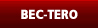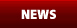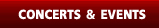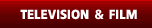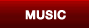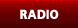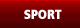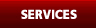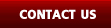Ó╣äÓĖĪÓ╣łÓĖĪÓĖĄÓ╣āÓĖäÓĖŻÓĖŁÓĖóÓĖ╣Ó╣łÓĖĢÓĖ▒ÓĖ¦ÓĖäÓĖÖÓ╣ĆÓĖöÓĖĄÓĖóÓĖ¦ Ó╣ĆÓĖŻÓĖ▓ÓĖĢÓ╣łÓĖ▓ÓĖćÓ╣ĆÓĖŖÓĖĘÓ╣łÓĖŁÓĖĪÓ╣éÓĖóÓĖćÓĖ¢ÓĖČÓĖćÓĖüÓĖ▒ÓĖÖÓĖ£Ó╣łÓĖ▓ÓĖÖÓĖäÓĖ¦ÓĖ▓ÓĖĪÓĖ¬ÓĖÖÓ╣āÓĖł ÓĖäÓĖ¦ÓĖ▓ÓĖĪÓĖäÓĖ┤ÓĖö ÓĖĢÓĖ▒ÓĖ¦ÓĖĢÓĖÖÓ╣üÓĖźÓĖ░Ó╣üÓĖŻÓĖćÓĖÜÓĖ▒ÓĖÖÓĖöÓĖ▓ÓĖźÓ╣āÓĖłÓĖéÓĖŁÓĖćÓĖüÓĖ▒ÓĖÖÓ╣üÓĖźÓĖ░ÓĖüÓĖ▒ÓĖÖ ÓĖäÓĖ¦ÓĖ▓ÓĖĪÓĖÜÓĖ▒ÓĖÖÓ╣ĆÓĖŚÓĖ┤ÓĖćÓ╣ĆÓĖŖÓĖĘÓ╣łÓĖŁÓĖĪÓ╣éÓĖóÓĖćÓĖ¢ÓĖČÓĖćÓĖüÓĖ▒ÓĖÖ ÓĖĪÓĖ▒ÓĖÖÓĖłÓĖĖÓĖöÓĖøÓĖŻÓĖ░ÓĖüÓĖ▓ÓĖóÓĖäÓĖ¦ÓĖ▓ÓĖĪÓĖĢÓ╣ēÓĖŁÓĖćÓĖüÓĖ▓ÓĖŻÓ╣āÓĖÖÓĖĢÓĖ▒ÓĖ¦Ó╣ĆÓĖŻÓĖ▓ ÓĖäÓĖ¦ÓĖ▓ÓĖĪÓĖĢÓ╣ēÓĖŁÓĖćÓĖüÓĖ▓ÓĖŻÓĖéÓĖŁÓĖćÓĖ×ÓĖ¦ÓĖüÓ╣ĆÓĖŻÓĖ▓Ó╣ĆÓĖŖÓĖĘÓ╣łÓĖŁÓĖĪÓ╣éÓĖóÓĖćÓ╣ĆÓĖŻÓĖ▓ÓĖ¢ÓĖČÓĖćÓĖüÓĖ▒ÓĖÖ Ó╣ĆÓĖŻÓĖ▓ÓĖ¬ÓĖŻÓ╣ēÓĖ▓ÓĖćÓĖ¬ÓĖŻÓĖŻÓĖäÓ╣īÓĖäÓĖ¦ÓĖ▓ÓĖĪÓĖÜÓĖ▒ÓĖÖÓ╣ĆÓĖŚÓĖ┤ÓĖć Ó╣ĆÓĖŻÓĖ▓ÓĖ¬ÓĖÖÓĖ▒ÓĖÜÓĖ¬ÓĖÖÓĖĖÓĖÖÓĖüÓĖ▓ÓĖŻÓ╣ĆÓĖŖÓĖĘÓ╣łÓĖŁÓĖĪÓ╣éÓĖóÓĖć ÓĖŚÓĖĖÓĖüÓĖüÓĖ▓ÓĖŻÓ╣ĆÓĖöÓĖ┤ÓĖÖÓĖŚÓĖ▓ÓĖćÓ╣ĆÓĖŻÓĖ┤Ó╣łÓĖĪÓĖłÓĖ▓ÓĖüÓĖüÓ╣ēÓĖ▓ÓĖ¦Ó╣ĆÓĖźÓ╣ćÓĖüÓ╣åÓ╣ĆÓĖ×ÓĖĄÓĖóÓĖćÓĖüÓ╣ēÓĖ▓ÓĖ¦Ó╣ĆÓĖöÓĖĄÓĖóÓĖ¦ ÓĖŚÓ╣ēÓĖ▓ÓĖŚÓĖ▓ÓĖóÓĖĢÓĖ▒ÓĖ¦Ó╣ĆÓĖŁÓĖćÓ╣āÓĖ½Ó╣ēÓ╣ĆÓĖĢÓĖ┤ÓĖÜÓ╣éÓĖĢ Ó╣āÓĖÖÓĖéÓĖōÓĖ░ÓĖŚÓĖĄÓ╣łÓĖäÓĖĖÓĖōÓĖŁÓĖŁÓĖüÓĖ£ÓĖłÓĖŹÓĖĀÓĖ▒ÓĖóÓ╣āÓĖÖÓĖüÓĖ▓ÓĖŻÓĖŚÓĖ▓ÓĖćÓ╣ĆÓĖöÓĖ┤ÓĖÖÓĖéÓĖŁÓĖćÓĖŖÓĖĄÓĖ¦ÓĖ┤ÓĖĢ Ó╣āÓĖŖÓ╣ēÓĖäÓĖ¦ÓĖ▓ÓĖĪÓĖøÓĖŻÓĖ▓ÓĖŻÓĖ¢ÓĖÖÓĖ▓Ó╣ĆÓĖøÓ╣ćÓĖÖÓ╣ĆÓĖéÓ╣ćÓĖĪÓĖŚÓĖ┤ÓĖ©ÓĖÖÓĖ│ÓĖŚÓĖ▓ÓĖć Ó╣üÓĖźÓĖ░Ó╣āÓĖŖÓ╣ēÓĖäÓĖ¦ÓĖ▓ÓĖĪÓĖØÓĖ▒ÓĖÖÓ╣ĆÓĖøÓ╣ćÓĖÖÓ╣üÓĖ£ÓĖÖÓĖŚÓĖĄÓ╣łÓĖŖÓĖĄÓĖ¦ÓĖ┤ÓĖĢ Ó╣üÓĖźÓ╣ēÓĖ¦ÓĖ½ÓĖ▒ÓĖ¦Ó╣āÓĖłÓĖłÓĖ░ÓĖÖÓĖ│ÓĖŚÓĖ▓ÓĖćÓĖäÓĖĖÓĖōÓ╣äÓĖøÓ╣ĆÓĖŁÓĖć ÓĖäÓĖ¦ÓĖ▓ÓĖĪÓĖŚÓ╣ēÓĖ▓ÓĖŚÓĖ▓ÓĖóÓĖüÓĖźÓĖ▓ÓĖóÓ╣ĆÓĖøÓ╣ćÓĖÖÓ╣ĆÓĖ×ÓĖĄÓĖóÓĖćÓĖŁÓĖĖÓĖøÓĖ¬ÓĖŻÓĖŻÓĖäÓ╣ĆÓĖźÓ╣ćÓĖüÓĖÖÓ╣ēÓĖŁÓĖóÓĖŚÓĖĄÓ╣łÓ╣ĆÓĖŻÓĖ▓ÓĖłÓĖ░ÓĖ£Ó╣łÓĖ▓ÓĖÖÓ╣äÓĖøÓ╣äÓĖöÓ╣ē Ó╣ĆÓĖĪÓĖĘÓ╣łÓĖŁÓĖäÓĖĖÓĖōÓĖĪÓĖĄÓ╣üÓĖŻÓĖćÓĖÜÓĖ▒ÓĖÖÓĖöÓĖ▓ÓĖźÓ╣ĆÓĖøÓ╣ćÓĖÖÓĖ£ÓĖ╣Ó╣ēÓĖŖÓ╣łÓĖ¦ÓĖóÓĖÖÓĖ│ÓĖŚÓĖ▓ÓĖć ÓĖ£ÓĖźÓĖ▒ÓĖüÓĖöÓĖ▒ÓĖÖÓĖĢÓĖ▒ÓĖ¦Ó╣ĆÓĖŁÓĖć ÓĖäÓĖĖÓĖōÓĖŁÓĖ▓ÓĖłÓĖłÓĖ░ÓĖŻÓĖ╣Ó╣ēÓĖ¬ÓĖČÓĖüÓĖøÓĖźÓĖŁÓĖöÓĖĀÓĖ▒ÓĖóÓĖ¢Ó╣ēÓĖ▓Ó╣ĆÓĖźÓĖĘÓĖŁÓĖüÓĖŚÓĖ│Ó╣āÓĖÖÓĖ¬ÓĖ┤Ó╣łÓĖćÓĖŚÓĖĄÓ╣łÓ╣ĆÓĖŻÓĖĄÓĖóÓĖÜÓĖćÓ╣łÓĖ▓ÓĖó Ó╣üÓĖĢÓ╣łÓĖÜÓĖ▓ÓĖćÓĖäÓĖŻÓĖ▒Ó╣ēÓĖćÓĖäÓĖ¦ÓĖ▓ÓĖĪÓĖ¬ÓĖĖÓĖéÓĖŚÓĖĄÓ╣łÓĖäÓĖĖÓĖōÓ╣āÓĖØÓ╣łÓĖ½ÓĖ▓ÓĖŁÓĖ▓ÓĖłÓĖ½ÓĖ▓ÓĖ×ÓĖÜÓ╣äÓĖöÓ╣ēÓ╣āÓĖÖÓĖŚÓĖĄÓ╣łÓ╣åÓ╣ĆÓĖ¬ÓĖĄÓ╣łÓĖóÓĖćÓĖŁÓĖ▒ÓĖÖÓĖĢÓĖŻÓĖ▓ÓĖóÓĖŚÓĖĄÓ╣łÓĖ¬ÓĖĖÓĖöÓĖüÓ╣ćÓ╣äÓĖöÓ╣ē Ó╣āÓĖÖÓ╣üÓĖĢÓ╣łÓĖźÓĖ░ÓĖ¦ÓĖ▒ÓĖÖ ÓĖźÓĖŁÓĖćÓĖŚÓĖ│Ó╣āÓĖÖÓĖ¬ÓĖ┤Ó╣łÓĖćÓĖŚÓĖĄÓ╣łÓĖäÓĖĖÓĖōÓ╣äÓĖĪÓ╣łÓĖüÓĖźÓ╣ēÓĖ▓ÓĖŚÓĖ│ÓĖöÓĖ╣
"; exit; } ?>

Room39
Mon 10/06/2013
Event Bridgestone road show @ Central Plaza Pattaya

Flure
Wed 12/06/2013
Pub Brick Bar @ ÓĖ¢ÓĖÖÓĖÖÓĖéÓ╣ēÓĖ▓ÓĖ¦ÓĖ¬ÓĖ▓ÓĖŻ

Gail TGT
Thu 13/06/2013
Event Milk Fesitval 2013 @ The Mall ÓĖÜÓĖ▓ÓĖćÓ╣üÓĖä

Stamp
Thu 13/06/2013
Pub ÓĖŻÓ╣ēÓĖ▓ÓĖÖ ÓĖø1/2 @ ÓĖł.ÓĖĢÓĖŻÓĖ▓ÓĖö

Armchair
Fri 14/06/2013
Pub U Bar @ Ó╣éÓĖäÓĖŻÓĖ▓ÓĖŖ

Sugar eyes
Sun 16/06/2013
Event Doraemon Event 2013 @ Ó╣ĆÓĖŗÓ╣ćÓĖÖÓĖŚÓĖŻÓĖ▒ÓĖź ÓĖ×ÓĖ┤ÓĖ®ÓĖōÓĖĖÓ╣éÓĖźÓĖüOur Official Hotel PartnerÓĖüÓĖ┤ÓĖłÓĖüÓĖŻÓĖŻÓĖĪÓ╣ĆÓĖ×ÓĖĘÓ╣łÓĖŁÓĖüÓĖ▓ÓĖŻÓĖüÓĖĖÓĖ©ÓĖźÓ╣üÓĖźÓĖ░ Ó╣éÓĖäÓĖŻÓĖćÓĖüÓĖ▓ÓĖŻÓ╣ĆÓĖ×ÓĖĘÓ╣łÓĖŁÓĖ¬ÓĖ▒ÓĖćÓĖäÓĖĪ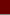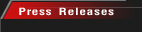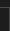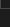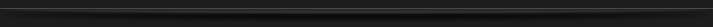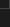Disclaimer: All information and content contained on these Web pages are based on the latest information available at the time of publication. BECTERO.COM reserves the right to change the content on this Web Site at any time without notice and shall not be responsible for any incorrectness, incompleteness, inappropriateness or typographical errors. Copyright ┬® 2008, www.bectero.com, All rights reserved.